# Algebraic systems, class of

(diff) ← Older revision | Latest revision (diff) | Newer revision → (diff)

A class of algebraic systems of the same type. All systems of a given type are assumed to be written in a given signatureand are called-systems. A classof-systems is called abstract if, whenever it contains a system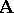, it also contains all-systems isomorphic to.

Let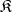be an abstract class of-systems. One says that an-systemhas a local set of-subsystems if there exists an inclusion-directed set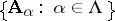of subsystems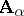of the systemwhich cover(i.e.) and which belong to. A classis called local if each-systemwith a local set of-subsystems belongs to the class. Theorems which establish the local nature of given abstract classes are called local (cf. Mal'tsev local theorems).

An-systemis called-approximable (or-residual) if, for any predicate(i.e. for any basic predicate as well as for the predicate coinciding with the equality relation in) and for any elements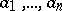infor which, there exists a homomorphism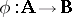of the systeminto some systemof the classfor which, again,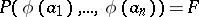. Any subsystem of a-approximable system is itself-approximable. Ifis the class of all finite-systems, a-approximable systemis called finitely approximable (or residually finite). If an abstract classhas a unit system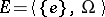, an-systemis-approximable if and only if it is isomorphically imbeddable in a Cartesian product of systems from the class. A classis called residual if all-approximable systems belong to the class. A classis homomorphically closed if it contains, for each of its-systems, also all-systems that are homomorphic images of. All residual homomorphically-closed classes are local .

A classof-systems is called (finitely) axiomatizable if there exists a (finite) setof first-order closed formulas of the signaturesuch thatconsists of exactly those-systems in which all formulas ofare true. Finitely-axiomatizable classes are also referred to as elementary classes. It has been shown  with the aid of the generalized continuum hypothesis that: 1) a classof algebraic systems is axiomatizable if and only if it is closed with respect to ultra-products, and its complement (in the class of all-systems) is closed with respect to ultra-powers; 2) a classof algebraic systems is elementary if and only if both it and its complement are closed with respect to ultra-products. The theory of axiomatizable classes of algebraic systems deals with the connection between the structural properties of these classes and the syntactic features of the formal language in which these classes may be specified. Axiomatizable classes which play an especially important role in algebra include varieties (cf. Algebraic systems, variety of) and quasi-varieties (cf. Algebraic systems, quasi-variety of), which are local and residual.

In addition to axiomatizability by first-order closed formulas, axiomatizability by special second-order closed formulas is also considered. To the function and predicate signature symbols(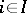),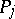(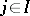) of a fixed signatureare added predicate variables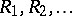. Let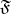be a quantifier-free formula of the first order, consisting of function and predicate signature symbols, predicate variables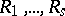and object variables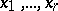. A second-order formula, where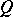is some sequence of quantifiers of the type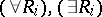or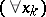is known as crypto-universal. The second-order formulas formed from the crypto-universal formulas without free object variables, with the aid of the logical connectivesand with subsequent quantification by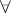on all free predicate variables encountered in the representations of the crypto-universal formulas, are called Boolean-universal formulas of the signature. A classof-systems is called quasi-universal if there exists a setof Boolean-universal formulas of the signaturesuch thatconsists of the-systems in which all formulas ofare true, and only of such systems. A quasi-universal class of-systems is local (Mal'tsev's theorem). A.I. Mal'tsev  gave a more detailed definition of a quasi-universal class.

How to Cite This Entry:
Algebraic systems, class of. Encyclopedia of Mathematics. URL: http://encyclopediaofmath.org/index.php?title=Algebraic_systems,_class_of&oldid=16625
This article was adapted from an original article by D.M. Smirnov (originator), which appeared in Encyclopedia of Mathematics - ISBN 1402006098. See original article